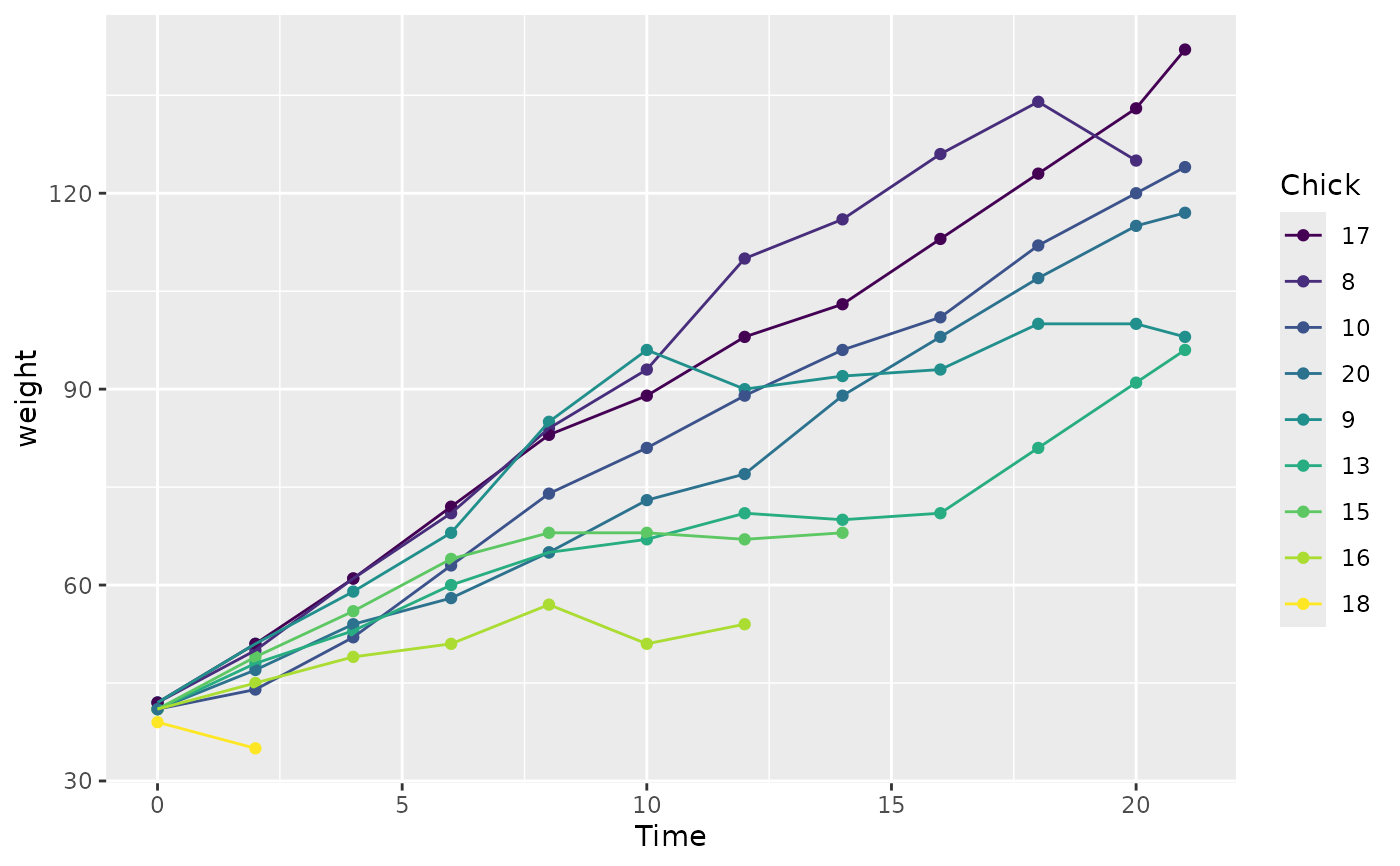fct_reorder() is useful for 1d displays where the factor is mapped to position; fct_reorder2() for 2d displays where the factor is mapped to a non-position aesthetic. last2() and first2() are helpers for fct_reorder2(); last2() finds the last value of y when sorted by x; first2() finds the first value.

## Usage

fct_reorder(
.f,
.x,
.fun = median,
...,
.na_rm = NULL,
.default = Inf,
.desc = FALSE
)

fct_reorder2(
.f,
.x,
.y,
.fun = last2,
...,
.na_rm = NULL,
.default = -Inf,
.desc = TRUE
)

last2(.x, .y)

first2(.x, .y)

## Arguments

.f

A factor (or character vector).

.x, .y

The levels of f are reordered so that the values of .fun(.x) (for fct_reorder()) and fun(.x, .y) (for fct_reorder2()) are in ascending order.

.fun

n summary function. It should take one vector for fct_reorder, and two vectors for fct_reorder2, and return a single value.

...

Other arguments passed on to .fun.

.na_rm

Should fct_reorder() remove missing values? If NULL, the default, will remove missing values with a warning. Set to FALSE to preserve NAs (if you .fun already handles them) and TRUE to remove silently.

.default

What default value should we use for .fun for empty levels? Use this to control where empty levels appear in the output.

.desc

Order in descending order? Note the default is different between fct_reorder and fct_reorder2, in order to match the default ordering of factors in the legend.

## Examples

# fct_reorder() -------------------------------------------------------------
# Useful when a categorical variable is mapped to position
boxplot(Sepal.Width ~ Species, data = iris)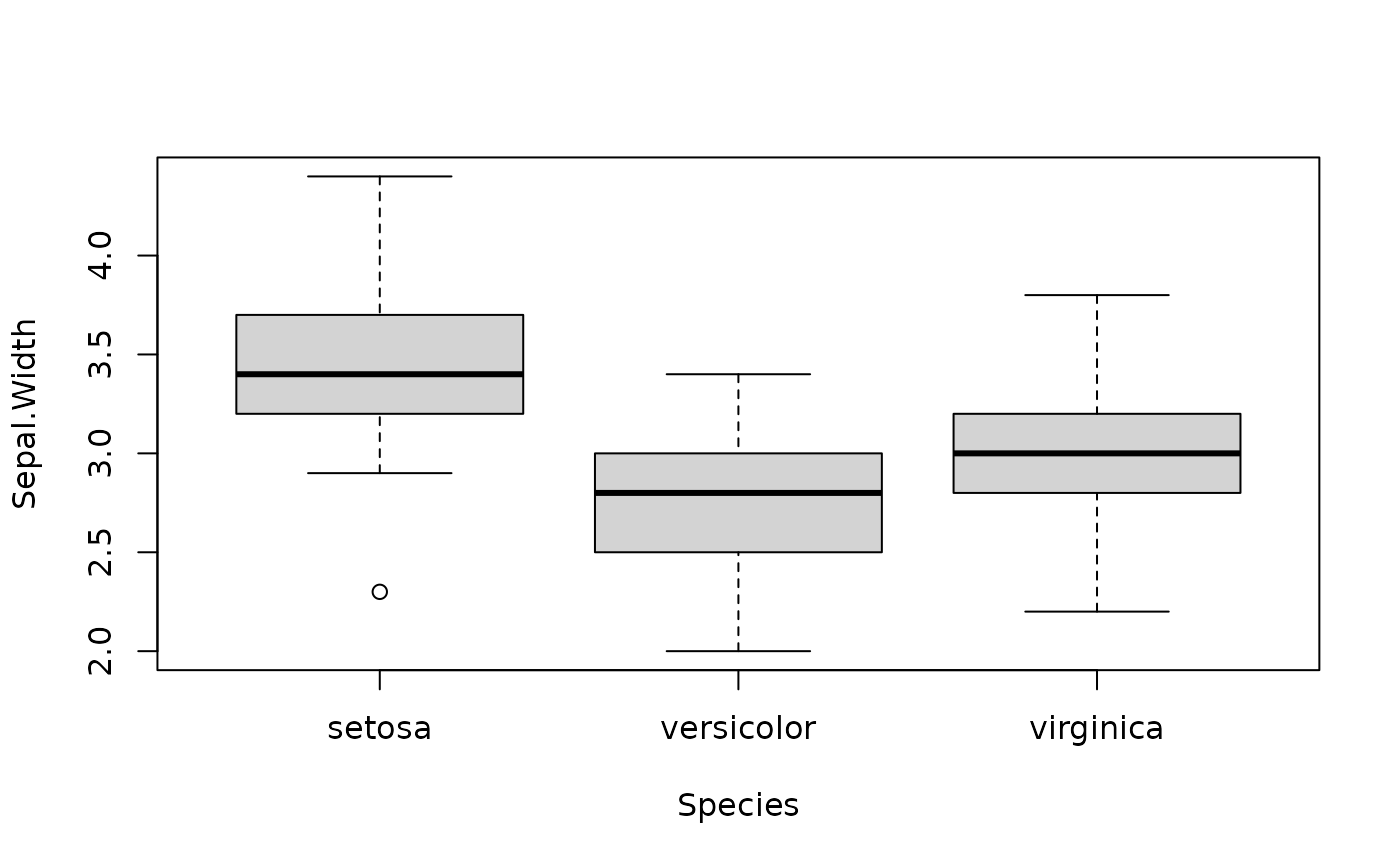boxplot(Sepal.Width ~ fct_reorder(Species, Sepal.Width), data = iris)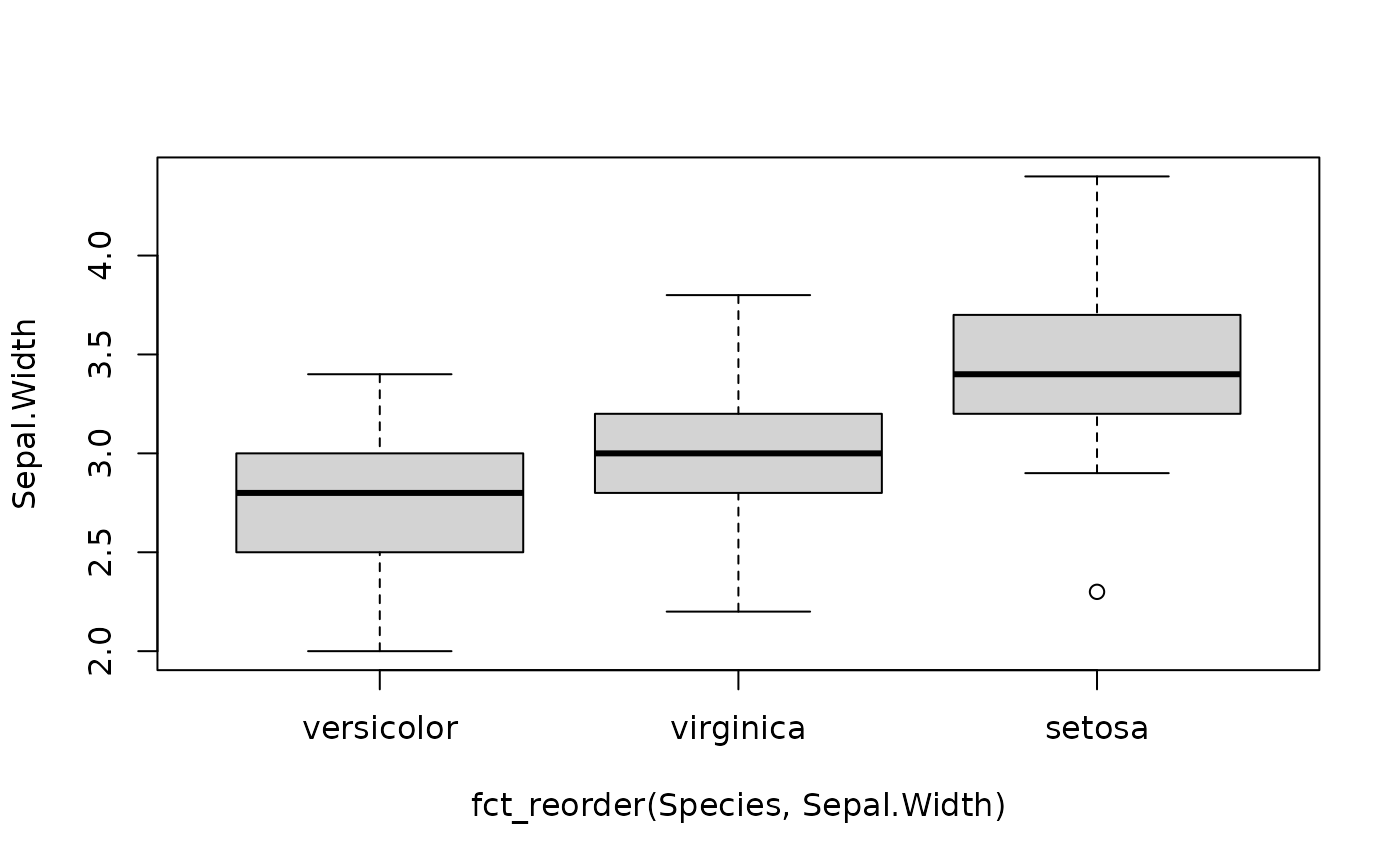# or with
library(ggplot2)
ggplot(iris, aes(fct_reorder(Species, Sepal.Width), Sepal.Width)) +
geom_boxplot()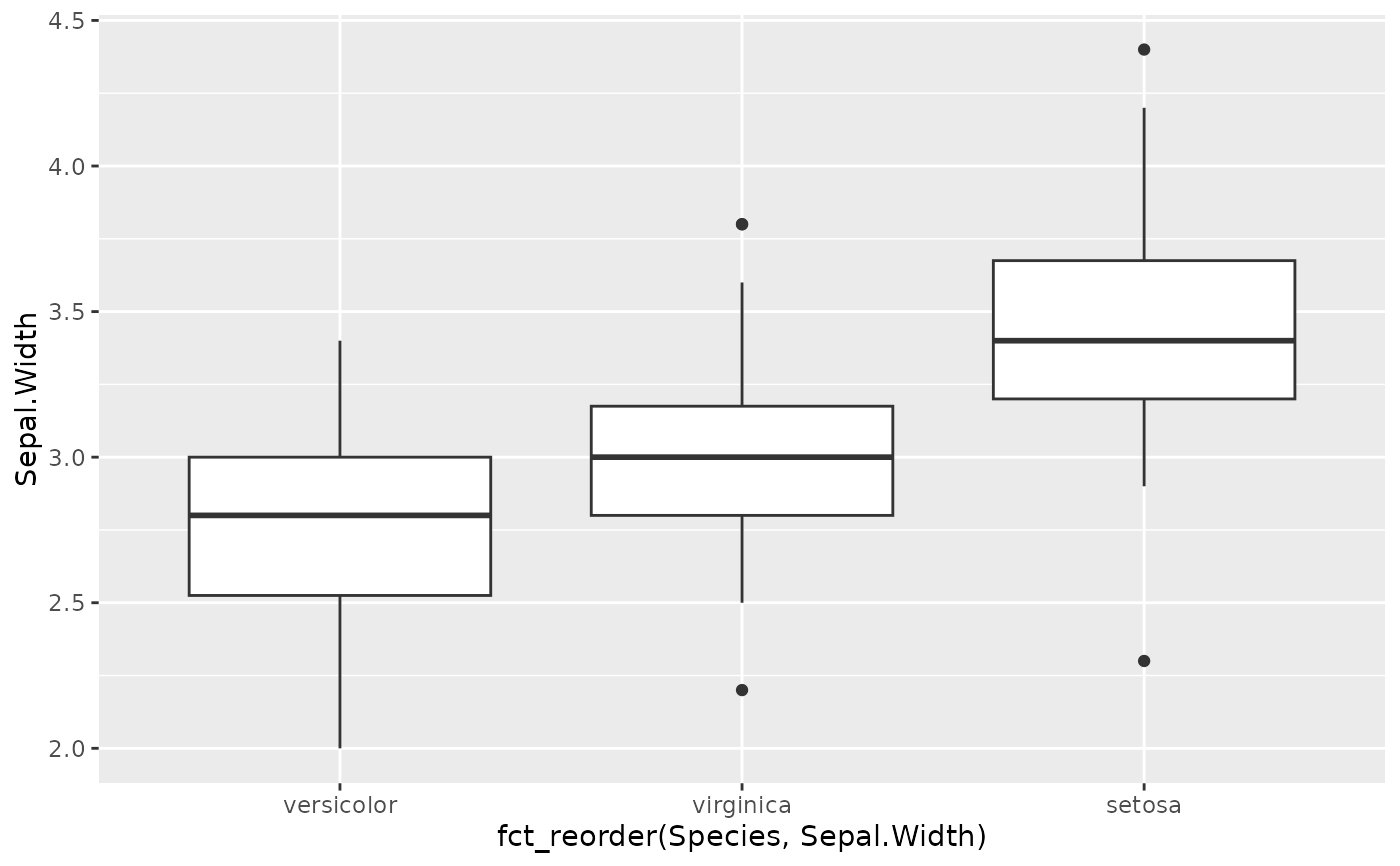# fct_reorder2() -------------------------------------------------------------
# Useful when a categorical variable is mapped to color, size, shape etc

chks <- subset(ChickWeight, as.integer(Chick) < 10)
chks <- transform(chks, Chick = fct_shuffle(Chick))

# Without reordering it's hard to match line to legend
ggplot(chks, aes(Time, weight, colour = Chick)) +
geom_point() +
geom_line()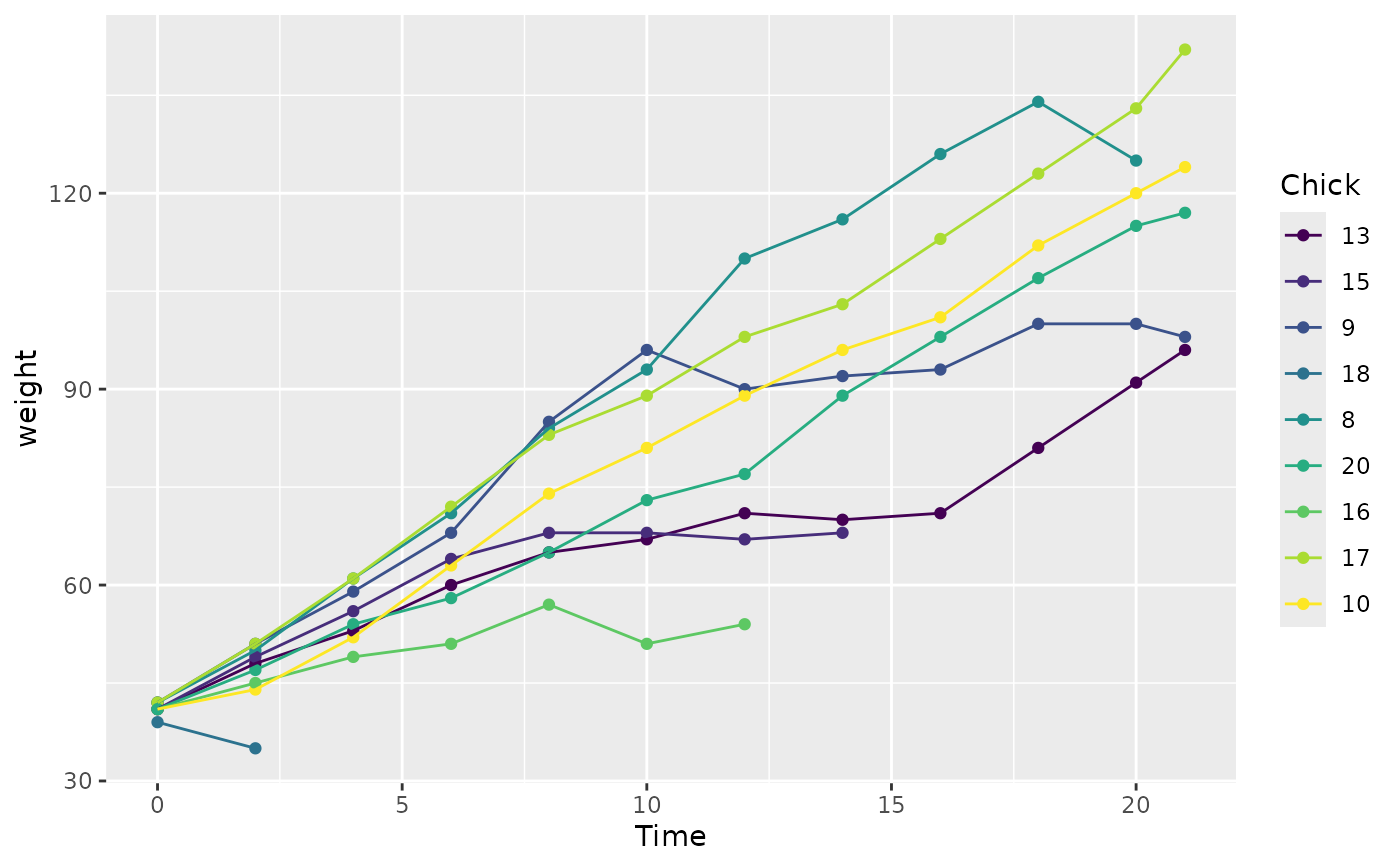# With reordering it's much easier
ggplot(chks, aes(Time, weight, colour = fct_reorder2(Chick, Time, weight))) +
geom_point() +
geom_line() +
labs(colour = "Chick")